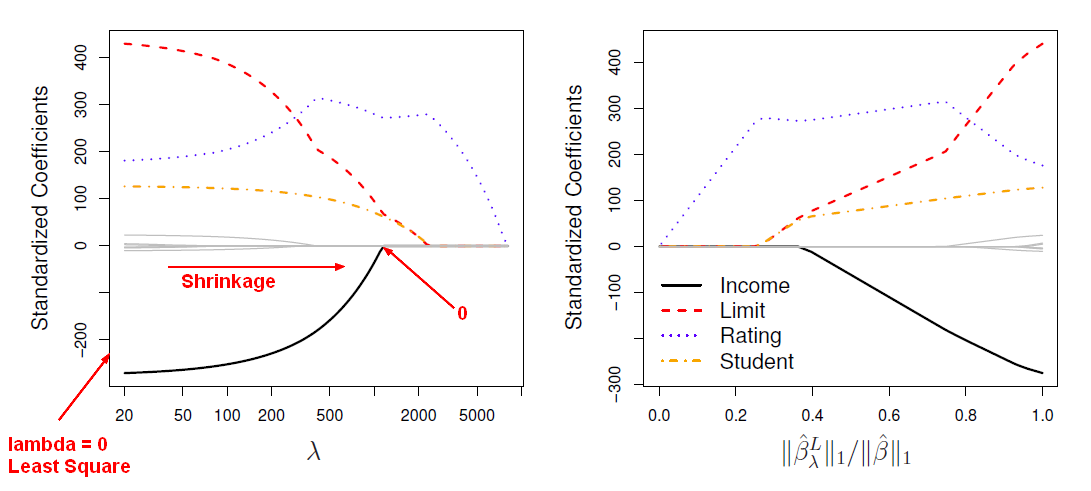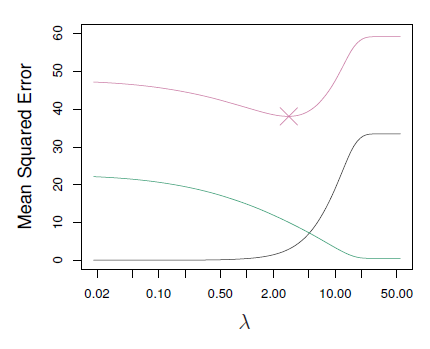# Statistics - Ridge regression

Ridge regression is a shrinkage method. It was invented in the '70s.

## Shrinkage Penalty

The least squares fitting procedure estimates the regression parameters using the values that minimize RSS.

In contrast, the ridge regression estimates the regression parameters $B$ minimizing the RSS with a penalty term (on the sum of squares of the coefficients)

$$\href{RSS}{RSS} + \underbrace{\lambda \sum^{\href{dimension}{P}}_{j=1}B^2_j}_\text{penalty term}} \\$$

So if lambda is big, the sum of squares of the coefficients must be small and will shrink the coefficients towards zero. And as lambda gets very big, the coefficients will all be zero.

The algorithm will try to make the RSS small but at the same time, the penalty term is going to push it in the other direction penalizing coefficients which get too large. The more non-zero a coefficient is, the larger the penalty term is. The larger the coefficients are, the bigger the penalty price is.

It's basically fit versus the size of the coefficients.

That penalty is called a shrinkage penalty because it's going to encourage the parameters to be shrunk toward 0.

## Tuning parameter $\lambda$

$$\overbrace{\underbrace{\lambda}_\text{Tuning parameter}} \sum^{\href{dimension}{P}}_{j=1}B^2_j}^\text{Penalty Term}}$$

The amount by which the coefficients are encouraged to be 0 is determined by the tuning parameter $\lambda$ .

If lambda is 0, this is only a least squares regression but the larger lambda is, the more and more a price will be payed for making these coefficients non-zero. If lambda is extremely large, the coefficients are going to be very close to 0 because they'll have to be close to 0 to make the penalty term small enough.

The penalty term has the effect of shrinking the coefficients towards 0 because if a coefficient is 0, the feature is not appearing in the model.

The size of the tuning parameter $\lambda$ is a trade-off decision between the fit versus the size of the coefficients. To be able to make this critical decision, the tuning parameter $\lambda$ will be chosen by resampling (namely cross-validation).

## Plot

### Standardized coefficients versus lambda

The below plot shows a ton of different models for a huge grid of lambda values.

On the left-hand side, lambda is close to 0, there's almost no constraint on the coefficients. This is the full least squares estimates. As lambda gets larger, it's pushing the coefficients towards 0 because we're paying more and more of a price for being non-zero. In the extreme, where lambda is a little more than 10,000, the coefficients are all essentially 0. In between, they're shrunken towards 0 as lambda gets larger, although not uniformly.

The red line indicates a value of lambda equals 100. For this lambda value, ridge regression chooses about four non-zero coefficients. At the red line:

• the B1 coefficient takes on a value of negative 100.
• B2 and B3 take on values of around 250.
• B4 takes on a value of around 100.
• The gray ones are basically essentially 0. They're not quite 0 but they are really small. They're close to 0. The coefficients are never exactly 0 unless you're extremely lucky. So ridge regression shrinks things in a continuous way toward 0 but doesn't actually select variables by setting a coefficient equal to 0 exactly whereas LASSO does.

### Standardized coefficient versus L2 norm

An equivalent picture than the previous one where the standardized coefficient is plotted as a function of a standardized l2 norm.

The standardized l2 norm is:

• the l2 norm of the least squares coefficient for a lambda
• divided by the l2 norm of the full least squares coefficient.

$$\frac{ \left \| \hat{\beta}^R_\lambda \right \|_2 }{ \left \| \hat{\beta} \right \| }$$

where $\hat{\beta}$ denotes the vector of least squares coefficient estimates

The notation $|| \beta ||_2$ denotes the l2 norm (pronounced “ell 2”) of a vector and is defined as:

$$l_2 \text{Norm} = || \beta ||_2=|| \beta_1 \dots \beta_2 ||_2 = \sqrt{\sum_{j=1}^P \beta^2_j}$$

On the left side of this picture, the coefficients are all basically 0, the lambda is very large and the l2 norm is 0. And on the right, lambda is very small, and we get the full least squares estimates. And in between, we get shrunken coefficients.

The x-axis goes from 0 to 1 because we're plotting as a function of a standardized l2 norm. At the right-hand side, the ridge regression estimate is the same as the least squares estimate, the numerator and denominator are the same, therefore the ratio is just 1.

## Prerequisites

#### Scale-variant

Standard least squares is scale-invariant but for penalized methods like ridge regression, the scaling does matter in an important way because the coefficients are all put in a penalty term together.

$$\lambda \sum_{j=1}^p \beta^2_j$$

If the units of variable are changed, it will change the scale of the coefficients. The units of the predictors affects the l2 penalty in ridge regression, and hence $\beta$ and $\hat{y}$ will both change

As a result, it's important to standardize the predictors before applying ridge regression.

### Lambda

The size of the tuning parameter $\lambda$ is a Bias-Variance trade-off decision between the fit versus the size of the coefficients. To be able to make this critical decision, the tuning parameter $\lambda$ will be chosen by resampling (namely cross-validation).

The above plot represents the bias, variance and test error as a function of lambda on the left and of the standardized l2 norm on the right where:

• the bias is the black line,
• the variance is the green line,
• the test error (MSE) is the purple line
• the horizontal dashed lines indicate the minimum possible MSE.
• The purple crosses indicate the ridge regression models for which the MSE is smallest.

When lambda gets larger, the bias is pretty much unchanged, but the variance drops. Therefore, by shrinking the coefficient toward 0, the ridge regression controls the variance.

Ridge regression doesn't allow the coefficient to be too big, and it gets rewarded because the mean square error, (which is the sum of variance and bias) is minimized and becomes lower than for the full least squares estimate.

### U-shaped curve

The U-shaped curve for the mean squared error (in purple) comes up again and again. Considering a bunch of different models that have different levels of flexibility or complexity, there is usually some sweet spot in the middle that has the smallest test error. And that's really what we're looking for.

## Documentation / Reference

Recommended PagesData Mining - Elastic Net Model

In statistics and, in particular, in the fitting of linear or logistic regression models, the elastic net is a regularized regression method that linearly combines the L1 and L2 penalties of the lasso...Statistical Learning - Lasso

Lasso is a shrinkage method. Ridge regression doesn't actually select variables by settings the parameters to zero. Lasso is a more recent technique for shrinking coefficients in regression that overcomes...Statistics - (Shrinkage|Regularization) of Regression Coefficients

Shrinkage methods are more modern techniques in which we don't actually select variables explicitly but rather we fit a model containingall p predictors using a technique that constrains or regularizes...